# Gabion Wall Design – Materials and Applications of Gabion WallReading time: 1 minute

## What is a Gabion Wall?

Gabion walls are gravity structures that are considerably flexible, environmentally friendly, cost effective, permeable, atheistically pleasing. It can be constructed as stepped front face or smoothed front face as shown in Figure 1.Figure 1: Different gabion wall face configuration, stepped face (left side) and smoothed face (right side)

The term Gabion is Latin and Italian word which means cage. The cages are filled with rocks and other construction materials and arranged to form the gabion wall. Steel wire baskets (cages) are produced in factory with 90x90x90 cm steel wire panel sides and the mesh size is 7.62 x 7.62 cm. It is unfolded at the site as shown in Figure 2 and different panel assembled to form the basket as shown in Figure 3.Fig: Types of Construction of a Gabion Wall

## Filling Materials of Gabion Walls:

Selection of rocks or stones is very important because the performance of the wall is based upon it. Gabion wall design depends on mass of filler materials and there are several factors that should be considered during filler selection such as:
• Grading of fill: it is substantially important because it helps to reduce small voids and consequently prevent settlement. Gabion graded fill between 100 mm to 200 mm is normal to use with nominal 6 percent smaller or larger diameter.
• Fill angularity: so important the fill angularity that increases interlocking and decreases face deformation of the wall. Rounded filler (rounded stone): possess lesser interlocking compared to angular rocks. Therefore, a stronger mesh might be used to compensate for that.
Two types of filler materials could be used for gabion wall in case of using costly filler. High cost filler at the face of the wall and low cost behind it.

## Design of Gabion Wall

Gabion wall is designed in the same way as gravity retaining wall. Units of gabion wall are considered as one cohesive mass for design purposes. The main forces acting on the wall are lateral earth pressure at the back face and vertical forces which is the weight of the wall as shown in Figure 4, the latter force is employed to resist the former one. Moreover, if other loads like seismic are come across, they should be taken into account in the analysis. Both stepped face and smoothed face gabion wall are designed depend on the same principle Figure 5 & Figure 6. Vertical load (Weight of unit length (1m)) = wall cross section x gabion fill density equation (1)Figure 4: Forces acting on gabion wallThe lateral earth pressure is computed according to coulomb equation: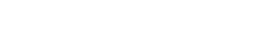Where: Pa: Total active force of triangular pressure distribution acting on the wall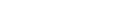H: Height of the wall Ka: Coefficient of active soil pressure, which can be obtained from the following equation:Where:Total active force (Pa) is inclined to the back face of the wall. Horizontal component is driving force which can be found using the equation: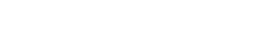Sliding resistance check Frictional resistance at the base of the wall resist against active earth pressure which tends to slide the wall. Fictional resistance can be obtained from the following equation: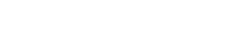Where:Overturning moment check Overturning is a type of failure that might happen as a result of active earth pressure force. Therefore, this should be prevented by resistant moment against overturning that is produced by the weight of the wall and other forces. Principle of static is used to calculate resistant moment (Mr) by taking moment about the toe of the wall.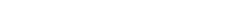Where: Mr: Resistance moment Sum (W x d): Sum of vertical forces (W) multiply by their distances (d) to the toe The resistance moment is calculated from the self-weight of the wall for the case where only the vertical forces are encountered and equation 6 will be: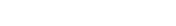à Equation (7) Where: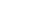: Self weight of gabion wall which vertically goes through the center of its cross sectional area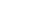: Distance from vertical forces to the wall toe. It can be achieved from taking moment about toe divided by total area: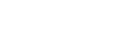The overturning moment (Mo) is obtained from active earth pressure which is perpendicular to the slope of back face and acts at H/3 from the base. In the presence of surcharge H/3 cannot be employed. The following equation is used to calculate distance from the base to the active earth pressure.So the overturning check is expressed as: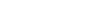Safety factor (SFs) against overturning is (2) Look at Figure 5 & Figure 6 to see different angles, forces and their distances on a typical gabion wall. Check bearing pressure: Compute eccentricity (e) of vertical force to determine whether resultant force is located within middle third of the base (B) or not: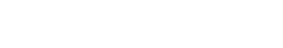Resultant force location will be within middle third if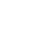The maximum pressure under the base is: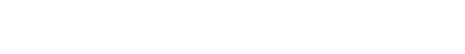## Applications of Gabion Walls

Generally applications of Gabion Walls are found in following cases:
• Retaining wall structures
• River and canal training